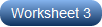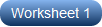# Multiplying Decimals by Powers of Ten Worksheets

Lead your crew through our printable multiplying decimals by powers of ten worksheets and solidify their understanding of place value. Help students in grade 5 and grade 6 comprehend that every time they multiply a decimal by a power of 10, its value increases. Explain patterns in the number of zeros of the product when multiplying a number by 10, 100, and 1000, and analyze the patterns in the placement of the decimal point. The trick, however, is to count the number of zeros in the power of 10 you are multiplying with, and that is the number of spaces you need to move the decimal point to your right. Watch the digits shift 1, 2, or 3 places to the left depending on the power of ten it is multiplied by and see the change in the place value of the digits. Evaluate instantly with our answer key. Grab our free multiplying decimals by the powers of ten worksheet for a sneak peek.

Multiplying Decimals by 10, 100, and 1000

Instruct 5th grade learners to observe the patterns as they multiply the same decimal number by 10, 100, and 1000 in these pdfs and comprehend that the digits remain the same while their position relative to the decimal point changes.Multiplying Decimals by Powers of Ten

Opt for our multiplying decimals by the powers of tens worksheet pdfs for 6th grade students to fluently multiply the decimal numbers with the specified powers of tens and shift the decimal point to the right as many places as there are zeros.Related Worksheets